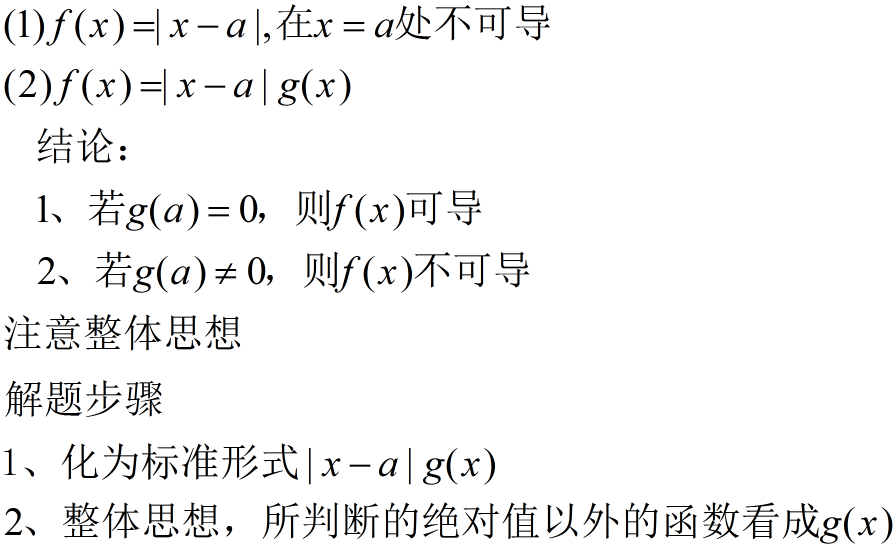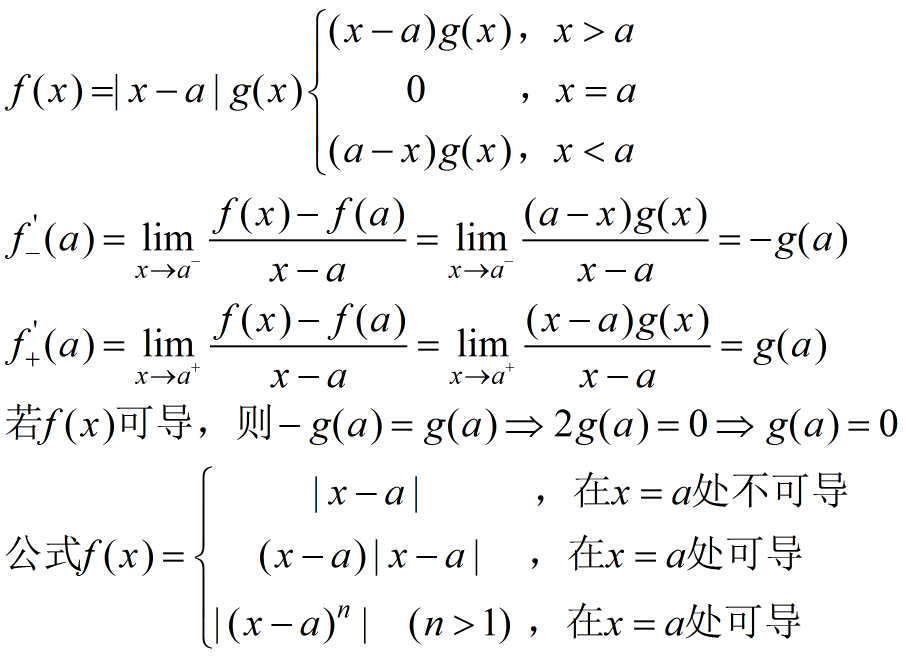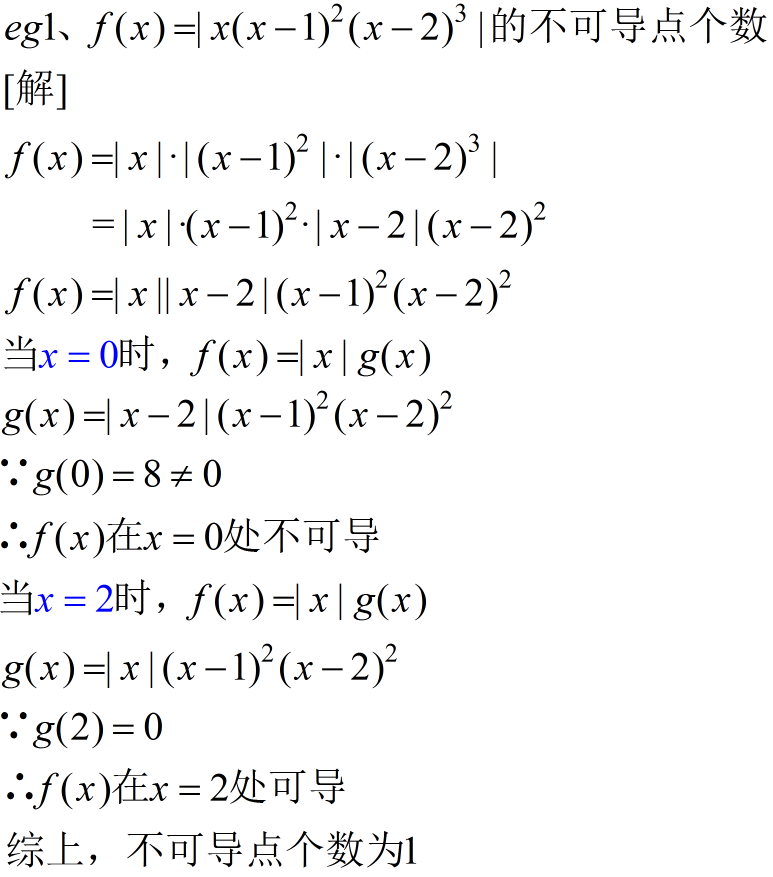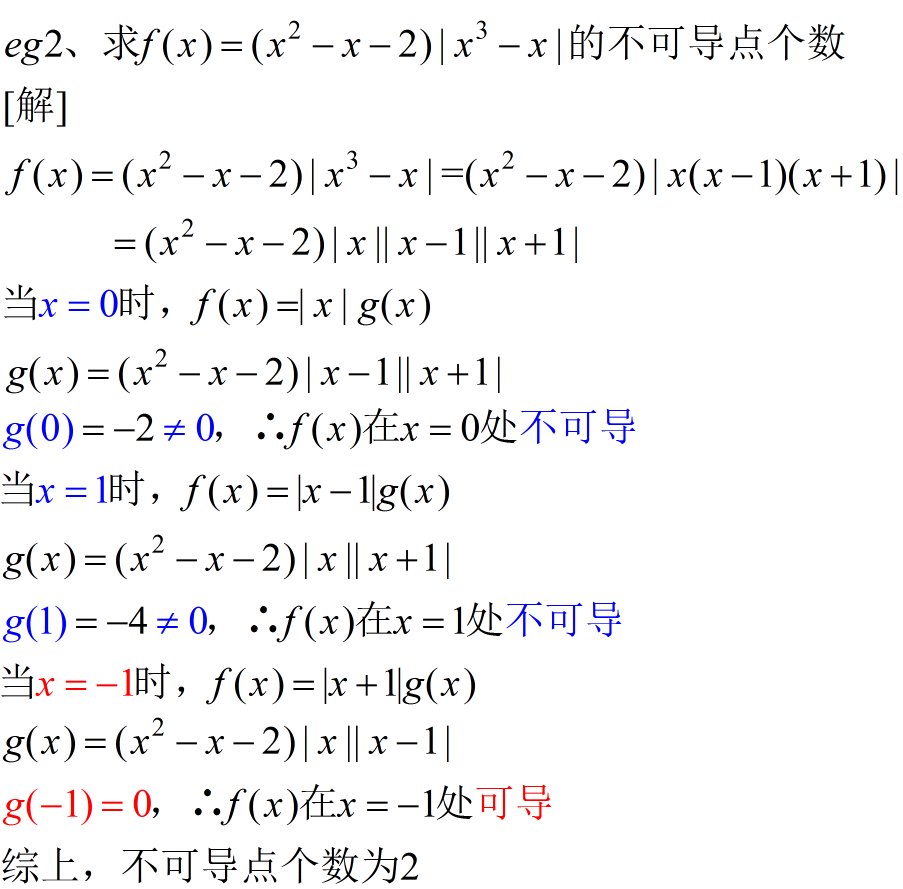• 可以学
虽然求可导点方法很多，但是我觉得这个方法是最简单最快捷的。 原创视频：<点这里>展开全文高等数学 期末考试
• 通过几例子指出判断函数在一点处是否可导要注意它的充分条件,剖析了错误判断产生的原因。
• 二阶可导点是拐点的必要条件 设存在，且点 （x0，f(x0) )为曲线拐点，则 判断拐点的第充分条件 设 f(x) 在 x=x0 处连续，在点 x=x0 的某去心邻域内二阶导数存在，且在该点的左右邻域内变号 则点 （x0，f(x0)...
二阶可导点是拐点的必要条件
设  存在，且点 （x0，f(x0) ) 为曲线拐点，则

判断拐点的第一充分条件
设 f(x) 在 x=x0 处连续，在点 x=x0 的某去心邻域  内二阶导数存在，且在该点的左右邻域内  变号
则点 （x0，f(x0) ) 为曲线拐点

判断拐点的第二充分条件
设 f(x) 在 x=x0 的某邻域内三阶可导，且  ,则点 （x0，f(x0) ) 为曲线拐点

判断拐点的第三充分条件
设 f(x) 在 x=x0 处 n 阶可导，且

当 n 为奇数时，点 （x0，f(x0) ) 为曲线拐点

证明：
由于n为奇数，令 n=2k+1，构造极限

上述洛必达法则成立的依据是最后的结果存在.
由函数极限的局部保号性可得：

同理可证  的情况
故点（x0，f(x0) ) 为曲线拐点
证毕

展开全文• 一阶可导点是极值点的必要条件 设 f(x) 在 x=x0 处可导，且在点 x0 处取得极值，则必有 判断极值的第充分条件 设 f(x) 在 x=x0 处连续，且在 x0 的某去心邻域内可导 x0 极小值点 ...
一阶可导点是极值点的必要条件
设 f(x) 在 x=x0 处可导，且在点 x0 处取得极值，则必有

判断极值的第一充分条件
设 f(x) 在 x=x0 处连续，且在 x0 的某去心邻域  内可导
x0 极小值点x0 极大值点

判断极值的第二充分条件
设 f(x) 在 x=x0 处二阶可导，且
x0 极大值点  x0 极小值点

判断极值的第三充分条件
设 f(x) 在 x=x0 处 n 阶可导，且

当 n 为偶数时
x0 极大值点x0 极小值点
证明：
由于n为偶数，令 n=2k，构造极限

上述洛必达法则成立的依据是最后的结果存在.
由函数极限的局部保号性可得：

x0 极大值点

x0 极小值点
证毕


展开全文• 最近复习到高数的一元函数微分部分 ，可导和可微是两个特别重要也特别容易混淆的概念。所以简单记录一下，便于自己理解，仅供参考。 导数 从物理角度来说（牛顿是从物理学的角度发明出的微积分）某点的导数就是一个...
最近复习到高数的一元函数微分部分 ，可导和可微是两个特别重要也特别容易混淆的概念。所以简单记录一下，便于自己理解，仅供参考。
导数
从物理角度来说（牛顿是从物理学的角度发明出的微积分）某点的导数就是一个该点的瞬时变化率的问题。 几何意义上来说（莱布尼茨从数学角度发明出的微积分），某点的导数是曲线在该点处的切线的斜率。 从定义来看，导数在本质上是一个极限问题。f(x)在

x

0

x_0

处的导数

f

(

x

)

ˋ

\grave{f(x)}

为：

f

(

x

)

ˋ

=

lim

⁡

Δ

x

→

0

f

(

x

0

+

Δ

x

)

−

f

(

x

0

)

Δ

x

\grave{f(x)}=\lim_{\Delta x\to \ 0} \frac{f(x_0+\Delta x)-f(x_0)}{\Delta x}

若该极限存在， 就说明函数在该点可导。当然我们知道，函数极限也可能不存在，所以该点也就不可导了。
微分：
从数形结合的方式理解：设一正方形边长为x，当边长增加

Δ

x

\Delta x

,面积增加

Δ

S

=

(

x

+

Δ

x

)

2

−

x

2

=

2

x

Δ

x

−

Δ

x

2

\Delta S=(x+\Delta x)^2-x^2=2x\Delta x-\Delta x^2

当

Δ

x

→

0

{\Delta x\to \ 0}

时，

(

Δ

x

)

2

(\Delta x)^2

对于

Δ

x

\Delta x

来说是高阶无穷小,记为

o

(

Δ

x

)

o(\Delta x)

，可以看作误差， 忽略不计。

2

x

Δ

x

2x\Delta x

才是增量的主要部分，我们叫做主部。
推广至一般函数，对于函数

f

(

x

)

f(x)

在

x

0

x_0

的某领域内有定义，那么函数增量记为：

Δ

y

=

f

(

x

+

Δ

x

)

−

f

(

x

)

\Delta y=f(x+\Delta x)-f(x)

若存在与

Δ

x

\Delta x

无关的常数

A

A

使得

Δ

y

=

A

Δ

x

+

o

(

Δ

x

)

\Delta y=A\Delta x+o(\Delta x)

,那么称

f

(

x

)

f(x)

在

x

0

x_0

处可微，微分为

A

Δ

x

A\Delta x

，即增量的线性主部。记作

d

y

∣

x

=

x

0

=

A

Δ

x

dy\lvert _{x=x_0}=A\Delta x

。 （其实这里的常数

A

A

就是

f

(

x

)

f(x)

在该点处的导数） 又因为

Δ

x

=

d

x

\Delta x=dx

（这里要理解一下为什么

Δ

x

=

d

x

\Delta x=dx

其实严格意义上

Δ

x

=

d

x

\Delta x=dx

因为一个是增量，一个是微分。 根据上面微分的定义 我们可以知道：

d

x

=

A

Δ

x

+

o

(

x

)

dx=A \Delta x+o(x)

所以他们之间有一个无穷小的误差。我们忽略不计）

d

y

∣

x

=

x

0

=

A

Δ

x

=

y

(

x

)

ˋ

Δ

x

=

y

(

x

)

ˋ

d

x

dy\lvert _{x=x_0}=A\Delta x=\grave{y(x)} \Delta x=\grave{y(x)} dx

d

y

d

x

∣

x

=

x

0

=

y

(

x

)

ˋ

d

x

d

x

=

y

(

x

)

ˋ

\frac{dy}{dx}\lvert _{x=x_0}=\frac{\grave{y(x)} dx}{dx}=\grave{y(x)}

通过上面的两个公式我们可以推出一元函数 可微必可导 两者互为充要条件。所以判断

f

(

x

)

f(x)

在

x

0

x_0

处是否可微就可以转换成是否可导的问题。 关于可微的含义：我们用线性增量

A

Δ

x

A \Delta x

代替复杂增量

Δ

y

\Delta y

,误差为

Δ

y

−

A

Δ

x

=

o

(

x

)

\Delta y - A\Delta x=o(x)

无穷小，可忽略不计。
几何意义：若

f

(

x

)

f(x)

在

x

0

x_0

处是否可微，则在

（

x

0

,

y

0

)

（x_0,y_0)

处可以用切线段(线性增量

A

Δ

x

A\Delta x

）近似替代曲线段（实际复杂增量

Δ

y

\Delta y

）。这个和后面函数在某个定义域内的积分的几何意义联系起来了，也是用线段近似替代曲线。
展开全文• ## 复合函数的可导性

千次阅读 2020-09-04 09:00:33
看内部函数的0的两方向趋向（并且在求导点的邻域不能有零值）， 并且内部函数与分母h的函数同阶（右侧不能为零，为零的话就可能不可导了，无穷时也不行，注意是充要条件） D项构造在零点分段的函数，从而导出...
• 在高等数学一元函数微分学中研究的关键问题之可导和可微，夹杂着函数连续，简短等知识点，这几相关的概念混在块总是难以理解，什么可导一定微，可导一定连续之类的。 这里把这几概念就自己的理解做一下...
• 4，如果二元函数f(x,y)的偏数fx(x,y),fy(x,y)在点(x0,y0)连续，如果二元函数f(x,y)的偏数f_x(x,y),f_y(x,y)在点(x_0,y_0)连续，如果二元函数f(x,y)的偏数fx​(x,y),fy​(x,y)在点(x0​,y0​)连续， ...
• ## 判断函数凹凸性

千次阅读 2020-05-18 17:39:37
假设fff微，则函数fff是凸函数的充要条件是domfdom fdomf是凸集且对于任意x,y∈domfx, y∈domfx,y∈domf, f(y)⩾f(x)+∇f(x)T(y−x)f(y) \geqslant f(x)+\nabla f(x)^{T}(y-x)f(y)⩾f(x)+∇f(x)T(y−x)成立。...算法
• 但是,函数在一点处解析与在一点处可导是不等价的概念. 即函数在一点处可导, 不一定在该点处解析. 但是,函数在一点处解析与在一点处可导是不等价的概念. 即函数在一点处可导, 不一定在该点处解析. 但是,函数在一点处...
• 二元函数凹凸性判断 二元函数凹凸性判断： 设f(x,y)f(x,y)f(x,y)在区域DDD上具有二阶连续偏数，且分别记为：A=fxx′′(x,y)，B=fxy′′(x,y)，C=fyy′′(x,y)A=f_{xx}^{''}(x,y)，B=f_{xy}^{''}(x,y)，C=f_{yy}^{'...机器学习 数据挖掘
• 1.f(x)n阶导函数存在 <=======> f(n)(x)存在 指的是在某个区间内有定义 2.f(x)n阶可导根据题意可以有两种不同的解释： 　①.题目中说的是在某点即在x=x0处n阶可导，指的是f(n)(x0)存在。 　②.题目直接说...
• 求导会发现这个函数一阶导数和二阶导数均为零 回顾几重要定理 这定理需要判断一阶导数左右符号 这定理不需要判断极值点附件的情况。 判别极值得第三充分条件 快速判别方法，将原函数中ln(1+x)...
• 判断极值的第充分条件 判断极值的第二充分条件 判断极值的第三充分条件 设 f(x) 在 x=x0 处 n 阶可导，且 当 n 为偶数时 必须n为偶数。 证明： 由于n为偶数，令 n=2k，构造极限 上述洛必达法则成立的依据是...
• 、极值点 ...函数的拐点理解为导数的极值点，因此上述关于极值点的结论都“稍加改变”后用于判断拐点，下面是一些常用结论： 拐点的必要条件： 拐点的充分条件：且在左右两侧变号 利用三阶...
• 判断连续与可导的关系3.关于导数定义的证明题4.基本复合函数求导5. 基本高阶求导6. 抽象函数求导 1. 利用导数定义求极限 导数的两种定义 f′(x0)f'{(x_0)}f′(x0​) = lim⁡x→x0f(x)−f(x0)x−x0\lim_{x \to x_0} ...
• 函数极值点一定是驻点，驻点不一定是极值点，驻点为两数存在且均为0的称为驻点 令A=，B=，​​​​​C= 若AC-B^2>0，则为极值点，且A>0为极小值 若AC-B^2<0，则不是极值点 若AC-B^2=0，则进一步...
• 先看两小的结论，有点拗口： ... 第类间断点的函数仍然积，事实上，这句话的完整描述是：有限类间断点的函数积，但是只要是第类间断点，即使是去间断点，仍然不存在原函数。 换句话数学
•算法 高等数学
• 例如三维空间的▽f(x)是一个三维空间的向量，而▽f(x)⊥就是与这个向量内积为零的点，这些点组成了一个二维平面。   posted on 2018-12-26 19:52 CreatorKou 阅读(...) 评论(......
• 而偏连续则是更强的条件，即偏存在且连续可以推出多元函数连续，反之不。 下面来分析，首先大家需要了解这些定义都是人定义出来的，可以反映多元函数的部分特征。所以，只要掌握了这些定义的意义就可以看出其...
• 假设fff微，则函数fff是凸函数的充分必要条件是domfdom fdomf是凸集且对于任意x,y∈domfx,y\in dom fx,y∈domf，下式成立 f(y)≥f(x)+∇f(x)T(y−x)f(y)\geq f(x)+\nabla f(x)^T(y-x)f(y)≥f(x)+∇f(x)T(y−x) 下...
• 函数有原函数，是指有一个函数的导数等于这个函数，及存在一个可导函数，其导函数等于目标函数函数可积，是指如果f(x)在[a,b]上的定积分存在（即曲线和轴围出来的面积存在且不为无穷），则说f(x)在[a,b]上可积，即...
• 所以，当我们判断一个导函数在某点连不连续，就要看上面这个式子成立与否,通常情况下我们要判断函数都是连续的，因为不连续的情况太好判断了。 f(x)f(x)f(x)是连续函数，f’(a)=limx−>af(x)−f(a)x−a=limx−&...
• 机器学习之支持向量机（三）：核函数和KKT条件的理解注：关于支持向量机系列文章是借鉴大神的神作，加以自己的理解写成的；若对原作者有损请告知，我会及时处理。转载请标明来源。序：我在支持向量机系列中主要讲...
• ## 机器学习之凸函数的四种判断方法

千次阅读 多人点赞 2019-04-24 12:08:11
函数的最大便利性就是，再进行优化求解的时候，令一阶为零后，所求出的值必是全局极小值。 判断是否为凸函数有4种方法，如下图所示。（为了避免在电脑上打公式的繁琐，直接以图片的形式展示） 以下是参考《机器...机器学习
• 首先定义凸集，如果x，y属于某个集合M，并且所有的θx+(1-θ)f(y)也属于M，那么M为一个凸集。如果函数f的定义域是凸集，并且满足 f(θx+(1-θ)y)≤θf(x)+(1-θ)f(y) 则该函数为凸函数。 如果函数存在二阶并且为正...机器学习 算法 python 数学建模
• 如果一个矩阵是方阵，那它的迹tr(A)等于对角线的元素之和。 多元函数判断驻点性质的方法： 找到多元函数jacobian向量（即目标函数对自变量的一阶偏数向量）为0的那些点，即驻点； 将各驻点带入Hessian矩阵...驻点 梯度下降 最优化方法...